# Solutions for Practice session 2¶

### Exercise 1. K-nearest Neighbors¶

Consider the same classification task as in practice 1, however this time solve it using \textit{K-nearest neighbors algorithm}. As before, we are given data about 5 cats and 5 dogs. The cat training instances are $\{(4,2),(5,1),(5,2),(5,3),(6,2)\}$, and dog training instances are $\{(7,4),(11,8),(11,10),(13,8),(13,10)\}$ where the first element of the pairs correspond to the weight (in unit of $\textit{pettygrams}$) and second element to the height (in unit of $\textit{pettymeters}$).

(a) Consider a new instance at (6.0,3.5). Based on KNN, what would be the class with $K=\{1, 2, 3, 5, 10\}$

$x^i = (x^i_1, x^i_2)$ : instance i in our training data (for both cat/dog), i=[1,10]

$x' = (x'_1, x'_2)$: new instance (that we would like to find its label)

euclidean_distance = $\sqrt{(x^i_1-x'_1)^2 + (x^i_2-x'_2)^2}$

We write down distance of new $x'$ with $x^i$s in separate sets corresponding to each class as follows:

$dists_{cat} = \{\overbrace{\sqrt{6.25}}^\text{a}, \overbrace{\sqrt{7.25}}^\text{b}, \overbrace{\sqrt{3.25}}^\text{c}, \overbrace{\sqrt{1.25}}^\text{d}, \overbrace{\sqrt{2.25}}^\text{e}\}$

$dists_{dog} = \{\overbrace{\sqrt{1.25}}^\text{f}, \overbrace{\sqrt{25.25}}^\text{g}, \overbrace{\sqrt{67.25}}^\text{h}, \overbrace{\sqrt{69.25}}^\text{i}, \overbrace{\sqrt{91.25}}^\text{j}\}$

$k=1:$ there are two possibilities, either $\{d\}$ or $\{f\}$ So the best guess is randomly picking one

$k=2: \{d, f\}$ => 1 cat, 1 dog => tie, determine the class randomly!

$k=3: \{d, f, e\}$ => 2 cats, 1 dog => class is cat by majority

$k=5: \{d, f, e, c, b\}$ => 4 cats, 1 dog => class is cat by majority

$k=10: \{a, b, c, d, e, f, g, h, i\}$ => 5 cats, 5 dogs => tie, determine class randomly!

(b) Between odd and even values for K which one do you think should be preferred and Why?

Answer: We should choose odd values for K to avoid a tie. Even values for K are more likely to lead to a tie unless all elements within the vicinity of the new instance are from the same class.

(c) Visualize the decision boundary for K=1. Optionally, you can also try $K=\{2,3,5\}$ in case you have implemented the decision boundary visualization in Python.

Answer: For the visualization of the decision boundaries we use the code below:

In :
import pandas as pd
import numpy as np
from matplotlib import pyplot as plt


Optional styling:

In :
plt.style.use('seaborn-whitegrid') # Plot style
import seaborn as sns


#### Dataset¶

Define the dataset about cats and dogs and take a look at it

In :
data = pd.DataFrame({
'mass':   [4, 5, 5, 5, 6, 7, 11, 11, 13, 13], # in kittygrams
'height': [2, 1, 2, 3, 2, 4, 8,  10, 8,  10], # in doggometers
'label':  ['cat']*5 + ['dog']*5
},
columns = ['mass', 'height', 'label'] # mantains column order
)
data
new_instance = (6,3.5)


#### Plot¶

It is always useful to plot your data. We will mainly use matplotlib for that purpose.

We will plot the same data again during this session and it is a good practice to wrap up this piece of code into a function

In :
def plot_cats_vs_dogs(data):
# Specify figure parameters
fig, ax = plt.subplots(figsize=(7, 5.5))
# Data to plot
ax.plot(data.loc[data.label == 'cat', 'mass'], data.loc[data.label == 'cat', 'height'], 'bo', label='cats')
ax.plot(data.loc[data.label == 'dog', 'mass'], data.loc[data.label == 'dog', 'height'], 'ro', label='dogs')

# Specify legend

# Specify axes parameters
ax.set_xticks(list(range(0, 15)))
ax.set_yticks(list(range(1, 12)))
ax.set_xlim(0, 14)
ax.set_ylim(0, 11)

# Axis labels
ax.set_xlabel('Mass')
ax.set_ylabel('Height')

return ax

# Show the plot inline
plot_cats_vs_dogs(data)
plt.legend(markerscale=1, frameon=True)
plt.show()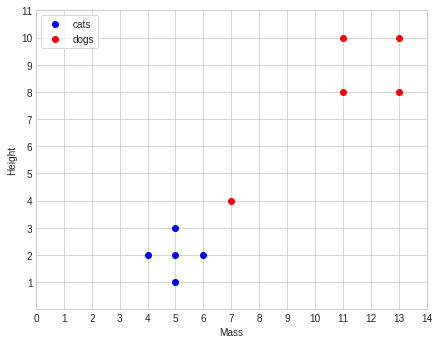#### KNN Algorithm¶

K-nearest neighbours algorithm makes decision for each point based on nearest neighbours' classes. We can visualize decision boundaries to compare classification with different K values.

In :
from sklearn.neighbors import KNeighborsClassifier
from sklearn.naive_bayes import GaussianNB

In :
def create_grid(X_train):
#     x = np.arange(min(X_train.x),max(X_train.x), (max(X_train.x)-min(X_train.x))/100 )
#     y = np.arange(min(X_train.y),max(X_train.y), (max(X_train.y)-min(X_train.y))/100 )
x = np.arange(0, 15, 0.1)
y = np.arange(0, 12, 0.1)
xx, yy = np.meshgrid(x, y)
X_grid = pd.DataFrame({'x':xx.flatten(),'y':yy.flatten()})
return X_grid

def plot_model(ax,X_train,y_train,X_grid,model):
y_pred = model.predict(X_grid)
X_grid[y_pred=='dog'].plot(x='x',y='y',kind='scatter',s=20,color='red',ax=ax, alpha=0.1, zorder=-1)
X_grid[y_pred=='cat'].plot(x='x',y='y',kind='scatter',s=20,color='blue',ax=ax, alpha=0.1, zorder=-1)
X_train[y_train=='dog'].plot(x='x',y='y',kind='scatter',s=40,color='pink',ax=ax, alpha=0.1, zorder=-1)
X_train[y_train=='cat'].plot(x='x',y='y',kind='scatter',s=40,color='lightblue',ax=ax, alpha=0.1, zorder=-1);
ax.plot(new_instance, new_instance,'.g',markersize=12,label='new instance')

In :
def plot_decision_boundaries(model, data):
X_train = data.iloc[:, :2]
X_train.columns = ['x', 'y']
y_train = data.label
ax = plot_cats_vs_dogs(data)
X_grid = create_grid(X_train)
model = model.fit(X_train, y_train)
plot_model(ax, X_train, y_train, X_grid, model)

In :
for k in [1,2,3,5]:
model = KNeighborsClassifier(n_neighbors=k)
plot_decision_boundaries(model, data)
plt.legend(markerscale=1, frameon=True, loc='upper left')
plt.title('{} neighbours'.format(k))
plt.show()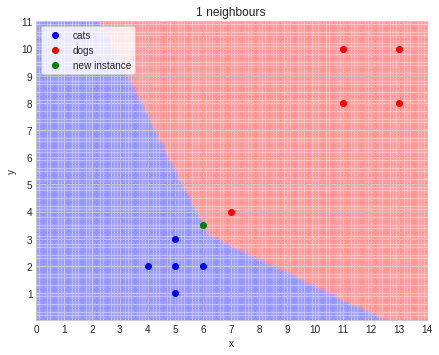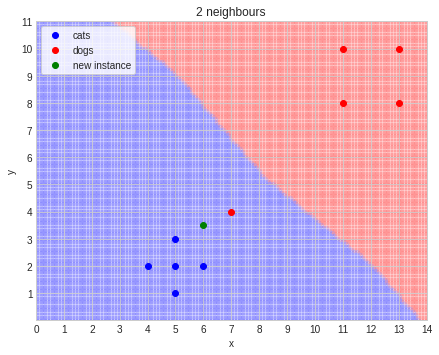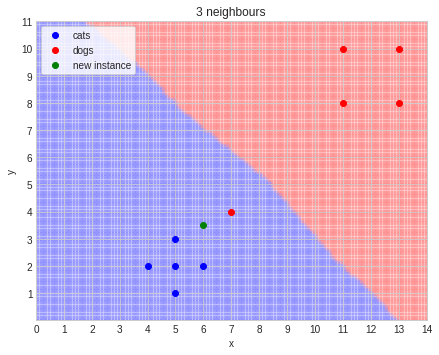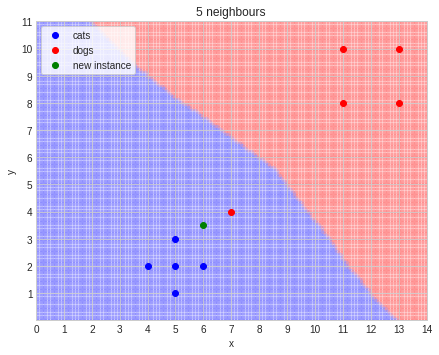### Exercise 2. Naive Bayes (Optional)¶

Complete the tasks based on table on the left (observations).

(a) Complete Frequency and Likelihood tables.

In :
from IPython.display import Image
Image(filename='freq_likelihood_table.png',width=800, height=400)

Out: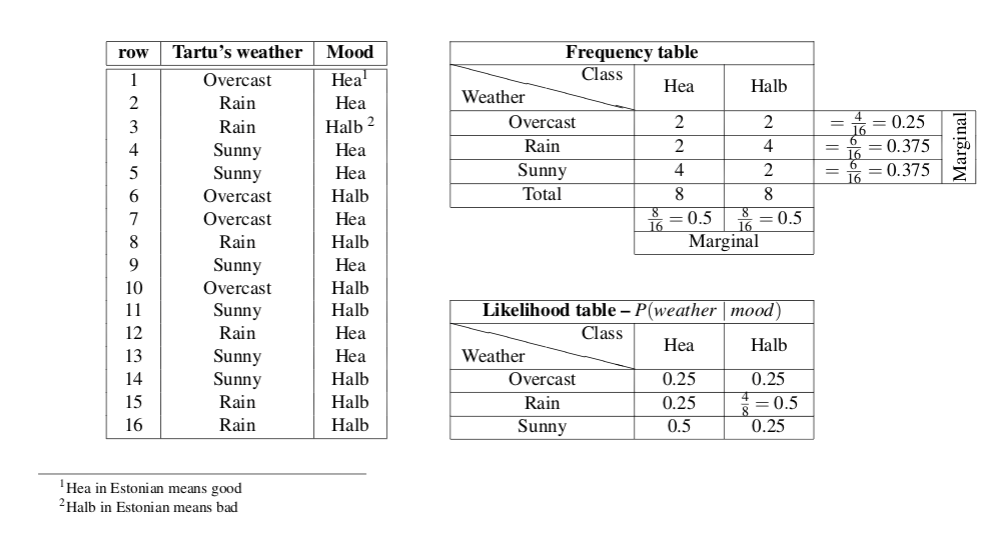(b) What is Naive Bayes Prediction for the mood when weather is Overcast, Rain, and Sunny respectively? Perform the necessary calculations.

Answer: For the sake of brevity we write $P(Hea\mid Overcast)$ instead of $P(Mood=Hea \mid weather=Overcast)$.

$P(mood \mid weather) = \overbrace{P(weather\mid mood)}^\text{Likelihood} \times \overbrace{P(mood)}^\text{Prior} / P(weather)$

P(weather) could be omitted as exists in both sides of the (in)equality. Since the value is positive it would be omitted by multiplying to the both sides of the (in)equality without changing the (in)equality.

$P(Overcast\mid Hea)P(Hea) = 0.25 \times 0.5 = 0.125 \quad\textbf{=}\quad P(Overcast\mid Halb)P(Halb) = 0.25 \times 0.5 = 0.125 \Longrightarrow \text{Hea/Halb}$

$P(Rain\mid Hea)P(Hea) = 0.25\times0.5 = 0.125 \quad\textbf{<}\quad P(Rain\mid Halb)P(Halb) = 0.5 \times 0.5 = 0.25 \Longrightarrow \text{Halb}$

$P(Sunny\mid Hea)P(Hea) = 0.5 \times 0.5 = 0.25 \quad\textbf{>}\quad P(Sunny\mid Halb)P(Halb) = 0.25 \times 0.5 = 0.125\Longrightarrow \text{Hea}$